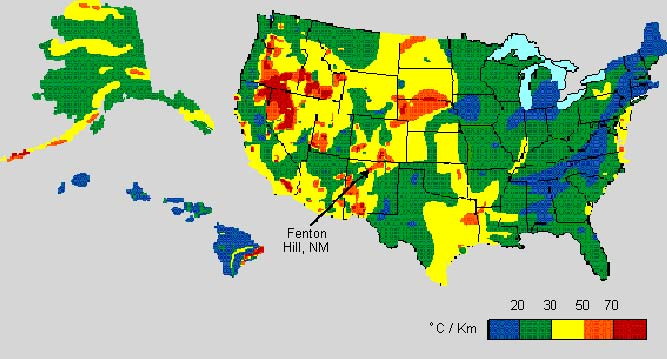Formation TEMPERATURE BASICS
Formation temperature is an essential piece of knowledge needed to calculate water resistivity, which in turn affects the calculation of water saturation and oil and gas in place. It may be measured with a continuous temperature log or during a drill stem or production test. The more usual method is to use the bottom hole temperature (BHT) recorded on the well log heading, along with an assumed surface temperature (SUFT), to create a temperature gradient graph. From this, temperature at any depth may be determined.

1: Grad = (BHT - SUFT) / BHTDEP
2: FT = SUFT + Grad * DEPTH

Where:
SUFT = surface temperature for temperature gradient (degrees Fahrenheit or Celsius)
BHT = bottom hole temperature (degrees Fahrenheit or Celsius)
Grad = temperature gradient (degrees F / foot or degrees C \ meter)
BHTDEP = depth at which BHT was measured (feet or meters)
DEPTH = mid-point depth of reservoir (feet or meters)
FT = formation temperature (degrees Fahrenheit or Celsius)

Many basins have local “hot spots”, with temperature gradients much higher than the basin average. Water sature will be too high and oil and gas inc place will be too low if a low temperature gradient or low formation temperature is used. instead of the correct one.ESTIMATING SURFACE TEMPERATURE
Temperature measurements specific to your area of interest are going to be sparse and you will have to do some searching for useful data. The map below gives a good idea of what to use for surface temperature (SUFT).Mean Annual Surface Temperature Map for North America - degrees F
The 40 deg F contour follows roughly along the US-Canada border except along the coast lines.
Contour interval is 5 deg F.ESTIMATING FORMATION TEMPERATURES
Temperature versus depth data from log headings can be plotted to estimate a best fit temperature gradient line. It doesn't have to be a straight line. See representative graphs below. In areas with sparse data, use the temperature gradient maps supplied below.Typical Depth - Temperature profiles. Create your own by best fit of BHT vs BHTDEP from data on log